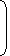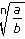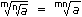# Tricks to solve surds and indices1. Laws of Indices:
1. am x an = am + n
2.  am = am – n an
3. (am)n = amn
4. (ab)n = anbn
5.an = an b bn
6. a0 = 1
2. Surds:Let a be rational number and n be a positive integer such that a(1/n) = aThen, a is called a surd of order n.
3. Laws of Surds:
1. a = a(1/n)
2. ab = a x b
3.= a b
4. (a)n = a
5.6. (a)m = am

Correct! Wrong!

Correct! Wrong!

Correct! Wrong!

Correct! Wrong!

#### (25)7.5 x (5)2.5 ÷ (125)1.5 = 5?

Correct! Wrong!

Don't miss out!
Subscribe To Our Newsletter

Learn new things. Get an article everyday.

Invalid email address
Give it a try. You can unsubscribe at any time.